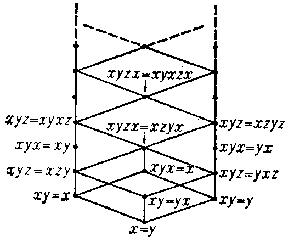# Idempotents, semi-group of

(diff) ← Older revision | Latest revision (diff) | Newer revision → (diff)

idempotent semi-group

A semi-group each element of which is an idempotent. An idempotent semi-group is also called a band (this is consistent with the concept of a band of semi-groups: An idempotent semi-group is a band of one-element semi-groups). A commutative idempotent semi-group is called a semi-lattice; this term is consistent with its use in the theory of partially ordered sets: If a commutative idempotent semi-group $S$ is considered with respect to its natural partial order, then $ab$ is the greatest lower bound of the elements $a,b\in S$. Every semi-lattice is a subdirect product of two-element semi-lattices. A semi-group $S$ is said to be singular if $S$ satisfies one of the identities $xy=x$, $xy=y$; in the first case $S$ is said to be left-singular, or to be a semi-group of left zeros, in the second case it is called right-singular, or a semi-group of right zeros. A semi-group is said to be rectangular if it satisfies the identity $xyx=x$ (this term is sometimes used in a wider sense, see ). The following conditions are equivalent for a semi-group $S$: 1) $S$ is rectangular; 2) $S$ is an ideally-simple idempotent semi-group (see Simple semi-group); 3) $S$ is a completely-simple semi-group of idempotents; and 4) $S$ is isomorphic to a direct product $L\times R$, where $L$ is a left-singular and $R$ is a right-singular semi-group. Every idempotent semi-group is a Clifford semi-group and splits into a semi-lattice of rectangular semi-groups (see Band of semi-groups). This splitting is the starting point for the study of many properties of idempotent semi-groups. Every idempotent semi-group is locally finite.

Idempotent semi-groups have been studied from various points of view, including that of the theory of varieties. The lattice of all subvarieties of the variety $\mathfrak V$ of all idempotent semi-groups has been described completely in ; it is countable and distributive, and every subvariety of $\mathfrak V$ is defined by one identity. See the figure for the diagram of this lattice; also indicated in this figure are the identities giving in $\mathfrak V$ the varieties on some of the lower "floors" .Figure: i050090a

How to Cite This Entry:
Idempotents, semi-group of. Encyclopedia of Mathematics. URL: http://encyclopediaofmath.org/index.php?title=Idempotents,_semi-group_of&oldid=31639
This article was adapted from an original article by L.N. Shevrin (originator), which appeared in Encyclopedia of Mathematics - ISBN 1402006098. See original article